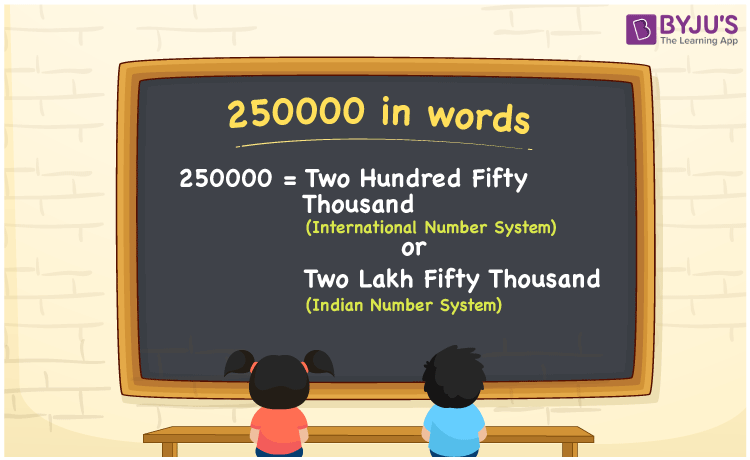# 250000 in Words

We can write 250000 in words as Two hundred Fifty thousand in the International number system and as Two lakh Fifty thousand in the Indian number system. In this article, you will learn how to convert the cardinal number 250000 to words of both International and Indian number systems.

 250000 in words Two hundred Fifty thousand (or) Two lakh Fifty thousand Two hundred Fifty thousand (or) Two lakh Fifty thousand in Numbers 250000

## 250000 in English Words

We generally write numbers in words using the English alphabet. Thus, we can read 250000 in English as “One thousand”.## How to Write 250000 in Words?

250000 in words – International number system

The below table depicts the place value chart for 250000 with respect to the International number system.

 Hundred thousands Ten thousands Thousands Hundreds Tens Ones 2 5 0 0 0 0

Here, ones = 0, tens = 0, hundreds = 0, thousands = 0, ten thousands = 5, hundred thousands = 2

2 × 100 thousand + 5 × 10 thousand

= 200 thousand + 50 thousand

= (200 + 50) thousand

= 250 thousand

Therefore, 250000 in words = 250 thousand = Two hundred Fifty thousand

250000 in words – Indian number system

Given below is the place value chart for 250000 in the Indian system.

 Lakhs Ten thousands Thousands Hundreds Tens Ones 2 5 0 0 0 0

Here, ones = 0, tens = 0, hundreds = 0, thousands = 0, ten thousands = 5, lakhs = 2

That means, 2 lakhs and 5 ten thousands

= 2 lakhs + (5 × 10) thousands

= 2 lakhs + 50 thousands

= Two lakh Fifty thousand

250000 is a natural number that precedes 250001 and succeeds 249999.

250000 in words – Two hundred Fifty thousand (or) Two lakh Fifty thousand

Is 250000 an odd number? – No

Is 250000 an even number? – Yes

Is 250000 a perfect square number? – Yes

What is the square root of 250000? – 500

Is 250000 a perfect cube number? – No

Is 250000 a prime number? – No

Is 250000 a composite number? – Yes

## Frequently Asked Questions on 250000 in Words

Q1

### How do you say 250000 in words?

We can say 250000 in words as Two hundred Fifty thousand (or) Two lakh Fifty thousand.
Q2

### How do you write Rs. 250000 in words on a cheque?

On a cheque, we write Rs. 250000 in words as “Two lakh Fifty thousand rupees only”.
Q3

### Write the answer in words for 135000 + 115000.

135000 + 115000 = 250000 Therefore, the value of 135000 + 115000 in words is Two hundred Fifty thousand (or) Two lakh Fifty thousand.